# Spherical functions

(diff) ← Older revision | Latest revision (diff) | Newer revision → (diff)

solid spherical harmonics, associated Legendre functions of the first and second kinds

Two linearly independent solutionsandof the differential equation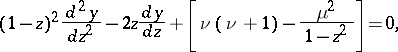whereandare complex constants, which arises in the solution of a class of partial differential equations by the method of separation of variables (cf. Separation of variables, method of). The points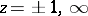are branching points of the solutions, in general. The spherical functions are particular cases of the hypergeometric functions (cf. Hypergeometric function):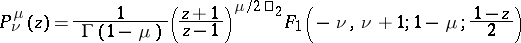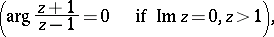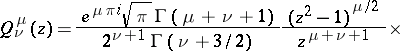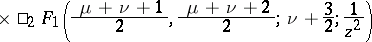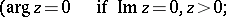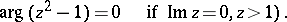The spherical functionsand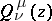are defined and single-valued in the domainsand, respectively, of the complex plane cut by the real axis from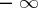to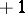.

If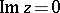,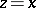,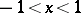, then the following functions are usually taken as solutions: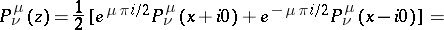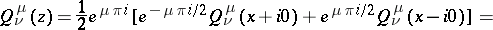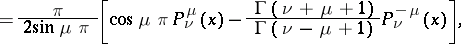where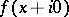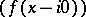are the values of the functionon the upper (lower) boundary of the cut.

When,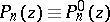are the Legendre polynomials. For zonal spherical functions see Spherical harmonics.

How to Cite This Entry:
Spherical functions. Encyclopedia of Mathematics. URL: http://encyclopediaofmath.org/index.php?title=Spherical_functions&oldid=16632
This article was adapted from an original article by Yu.A. BrychkovA.P. Prudnikov (originator), which appeared in Encyclopedia of Mathematics - ISBN 1402006098. See original article